# OpenCV 简介及其工程应用 - 游戏色块检测OpenCV 是一个基于 BSD 许可（开源）发行的跨平台的计算机视觉和机器学习软件库，可以运行在 Linux、Windows、Android 和 Mac OS 操作系统上。它轻量级而且高效——由一系列 C 函数和少量 C++ 类构成，同时提供了 Python、Ruby、MATLAB 等语言的接口，实现了图像处理和计算机视觉方面的很多通用算法。

OpenCV 在图像分割、人脸识别、物体识别、动作跟踪、动作分析、机器视觉等领域都有广泛的应用。

### 1. OpenCV 基本操作

#### 1.1 读取、显示以及保存操作

import cv2image = cv2.imread("test.jpg") # 读取操作cv2.imshow("test", image) # 显示操作cv2.waitKey() # 等待按键cv2.imwrite("save.jpg") # 保存操作

#### 1.2 改变色彩空间

image = cv2.imread("test.jpg")hsv = cv2.cvtColor(image, cv2.COLOR_BGR2HSV) # 转换到HSV空间hls = cv2.cvtColor(image, cv2.COLOR_BGR2HLS) # 转换到HLS空间lab = cv2.cvtColor(image, cv2.COLOR_BGR2Lab) # 转换到Lab空间gray = cv2.cvtColor(image, cv2.COLOR_BGR2GRAY) # 转换到GRAY空间（灰度图）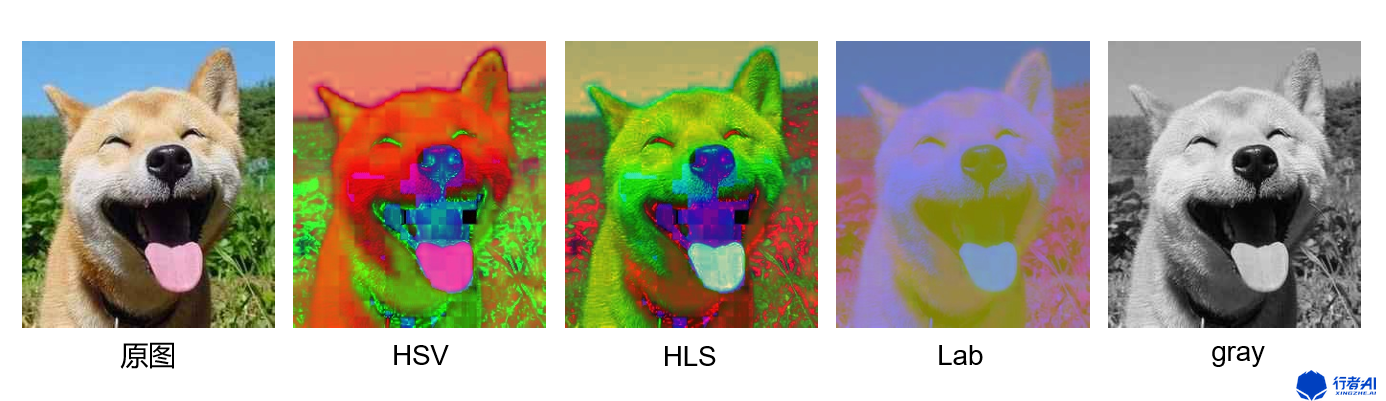HSV 这个模型中颜色的参数分别是：色调(H)，饱和度(S)，明度(V)，该模型常用来做绿幕分割。

#### 1.3 几何变换--缩放、平移、旋转

##### a. 缩放

image = cv2.imread("test.jpg")resize = cv2.resize(image, (), fx=0.5, fy=0.5, interpolation=cv2.INTER_AREA) # 长宽缩小到0.5倍

##### b. 平移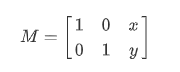import cv2import numpy as npimage = cv2.imread("test.jpg")rows, cols, channels = image.shapeM = np.float32([[1,0,100],[0,1,50]])res = cv.warpAffine(image, M, (cols, rows))

##### c. 旋转

image = cv2.imread('test.jpg')rows, cols, channels = image.shaperotate = cv2.getRotationMatrix2D((rows*0.5, cols*0.5), 45, 1) # 第一个参数：旋转中心点 第二个参数：旋转角度 第三个参数：缩放比例res = cv2.warpAffine(image, rotate, (cols, rows))

#### 1.4 平滑处理--模糊、滤波

image = cv2.imread('test.jpg')blur = cv2.blur(image, (5, 5)) # 均值滤波 第二个参数是卷积核大小median_blur = cv2.medianBlur(image, 5) # 中值滤波gaussian_blur = cv2.GussianBlur(image, (5, 5)) # 高斯模糊

#### 1.5 膨胀、侵蚀

##### a. 图像形态学操作

• 形态学有四个基本操作：侵蚀、膨胀、开、闭

• 膨胀与腐蚀是图像处理中最常用的形态学操作手段

• 膨胀就是图像中的高亮部分进行膨胀，“领域扩张”，效果图拥有比原图更大的高亮区域。侵蚀就是原图中的高亮部分被腐蚀，“领域被蚕食”，效果图拥有比原图更小的高亮区域

##### b. 膨胀与侵蚀

• 消除噪声

• 分割出独立的图像元素，在图像中连接相邻的元素

• 寻找图像中的明显的极大值区域或极小值区域

• 求出图像的梯度

image = cv2.imread("test.jpg")kernel = cv2.getStructuringElement(cv2.MORPH_RECT,(3, 3)) # 获取卷积核eroded = cv2.erode(image, kernel) # 腐蚀图像dilated = cv2.dilate(image, kernel) # 膨胀图像

##### c. 开运算和闭运算

• 开运算：先腐蚀后膨胀，用于移除由图像噪音形成的斑点

• 闭运算：先膨胀后腐蚀，用来连接被误分为许多小块的对象

#### 1.6 查找绘制轮廓

##### a. 查找轮廓

# opencv版本大于3contours, hierarchy = cv2.findContours(image, cv2.RETR_EXTERNAL, cv2.CHAIN_APPROX_NONE) # 第一个参数：查找的二值图像 第二个参数：轮廓检索模式 第三个参数：轮廓近似方法# 返回值contours为查找到的轮廓列表，hierarhy为轮廓之间的层级关系

##### b. 绘制轮廓

cv2.drawContours(temp,contours,-1,(0,255,0),3) # 第一个参数：画布，可以是原图 第二个参数：查找到的轮廓 第三个参数：-1表示全画 第四个参数：颜色 第五个参数：轮廓宽度

### 2. OpenCV 的工程应用-色块检测

#### 2.1 问题背景#### 2.2 问题分析#### 2.3 程序设计

##### a. 图像二值化

import cv2import numpy as npimage = cv2.imread("test.jpg")b, g, r = cv2.split(self.image) # 分离B、G、R通道b = np.where(b >= 250, 1, 0) # 找到G通道符合要求的像素点置1，不符合置0g = np.where(g >= 250, 1, 0) # 找到G通道符合要求的像素点置1，不符合置0r = np.where(r >= 250, 1, 0) # 找到G通道符合要求的像素点置1，不符合置0gray = b + g + r # 将三个通道叠加成一个通道gray = np.where(gray==3, 255, 0).astype(np.uint8) # 像素点为3的即为满足要求的点设置为白色，不符合设置为黑色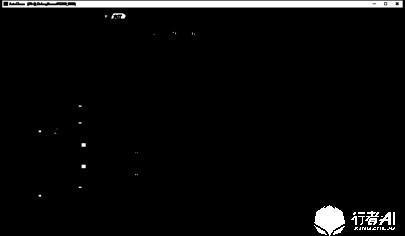##### b. 查找轮廓

contours, hierarchy = cv2.findContours(gray, cv2.RETR_EXTERNAL, cv2.CHAIN_APPROX_NONE) # 获取轮廓及层级关系##### c. 轮廓筛选

def screen_contour(contour):    contour_area = cv2.contourArea(contour)    if contour_area > self.area_limit:        return True    return Falsepass_contours = []for contour in contours:    if screent_contour(contour):        pass_contours.append(contour)

def screent_contour(contour):    width = np.max(contour[:,:,0]) - np.min(contour[:,:,0])    height = np.max(contour[:,:,1]) - np.min(contour[:,:,1])    block_area = width * heigh	contour_area = cv2.contourArea(contour) 	slimier = cv2.contourArea(contour) / block_area    if slimier > self.simliar_rate:       return True    return Falsepass_contours = []for contour in contours:    if screent_contour(contour):        pass_contours.append(contour)### 3. OpenCV 与矩阵

OpenCV 是如何完成以上这些操作的呢？

#### 3.1 图像读取

OpenCV 在读取图像的时候是将图像信息转换成了矩阵，默认矩阵为（height，width，channel)，channel 对应的是 B、G、R 通道，每个像素点的颜色由三个通道一起决定，和三元色的关系是一样的，B、G、R 的大小代表的是色彩比例。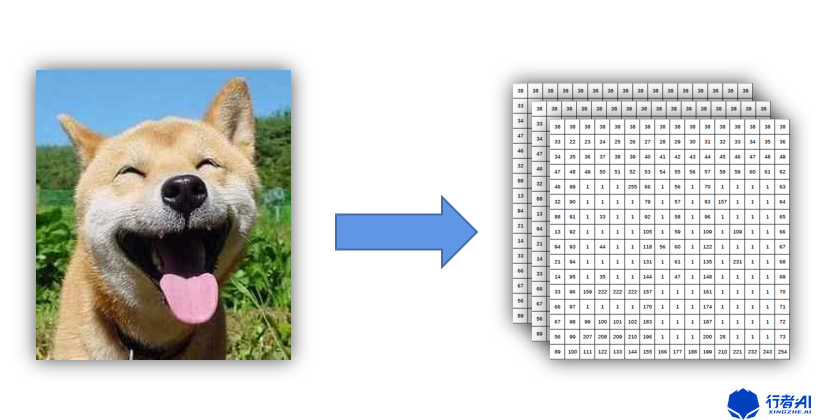#### 3.3 图像旋转的数学含义### 4. OpenCV 与机器学习

openCV 已经集成了很多机器学习算法、如 K 近邻(KNN)，支持向量机(SVM)、决策树、随机森林、Boost、逻辑回归、ANN 等。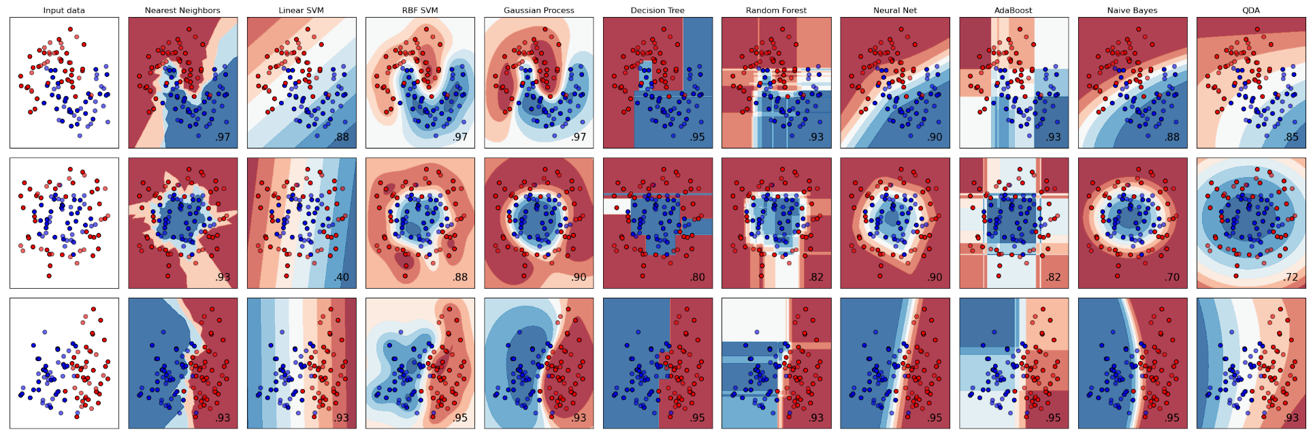### 5. 视频书籍推荐

#### 5.1 线性代数

3blue1brown《线性代数的本质》系列视频通过形象图像的动画直观的解释了线性代数的魔力，还有其它系列也很棒。

《程序员的数学 3 线性代数》也是一本很不错的线性代数教材，细细读完，受益匪浅。

#### 5.2 概率论

《程序员的数学 2 概率统计》很系统的讲解了概率统计的知识，不是很理解线性代数的话，推荐先看线性代数。

#### 5.3 OpenCV

《学习 OpenCV》是一本很不错的工具书。## 评论